ALL

Page: (Previous)   1  2  3  4  5  (Next)
ALL

### M

#### MAT-HS.A-CED.04

 MAT-HS Targeted Standards(A) Concept: Algebra(CED) Domain: Creating ExpressionsCluster: Create equations that describe numbers or relationships MAT-HS.A-CED.04 Rearrange formulas to highlight a quantity of interest, using the same reasoning as in solving equations. For example, rearrange Ohm’s law V = IR to highlight resistance R.

## Student Learning Targets:

• I can
• I can

• I can
• I can

### Skills (Performance) Targets

• I can solve for (isolate) a specific variable.
• I can perform transformations that produce equivalent equations (e.g., adding the same amount to both sides of the equation, etc.)
• I can solve multi-variable formulas or literal equations for a specific variable.

• I can
• I can

## Proficiency Scale

 Score Description Sample Activity 4.0 (advanced) In addition to Score 3.0, the student demonstrates in-depth inferences and applications regarding more complex material that go beyond end of instruction expectations. Solve for h:   3h+g=5h-hg 3.5 In addition to Score 3.0 performance, the student demonstrates in-depth inferences and applications regarding the more complex content with partial success. 3.0 (proficient) The student can: solve multi-variable formulas or literal equations for a specific variable. The student exhibits no major errors or omissions. Solve the following equation for b: A = 1/2h (b+c) Solve the following equation for a: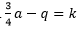Solve the following equation for b: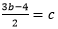2.5 The student demonstrates no major errors or omissions regarding the simpler details and processes (Score 2.0 content) and partial knowledge of the more complex ideas and processes (Score 3.0 content). 2.0 (progressing) There are no major errors or omissions regarding the simpler details and processes as the student can: identify appropriate inverse operations. solve a simple linear equation for y. However, the student exhibits major errors or omissions regarding the more complex ideas and processes. Solve the following equation for y: 2x - 3y = 8 1.5 The student demonstrates partial knowledge of the simpler details and processes (Score 2.0 content) but exhibits major errors or omissions regarding the more complex ideas and procedures (Score 3.0 content). 1.0 (beginning) With help, the student demonstrates a partial understanding of some of the simpler details and processes (Score 2.0 content) and some of the more complex ideas and processes (Score 3.0 content). 0.5 With help, the student demonstrates a partial understanding of some of the simpler details and processes (Score 2.0 content) but not the more complex ideas and processes (Score 3.0 content). 0.0 Even with help, the student demonstrates no understanding or skill.

## Resources

 Web Vocab literal equation

#### MAT-HS.A-REI

Domains are larger groups of related standards. So the Domain Score is a calculation of all the related standards. So click on the standard name below each Domain to access the learning targets and proficiency scales for each Domain's related standards.

# Reasoning with Equations and Inequalities

• Understand solving equations as a process of reasoning and explain the reasoning
• Solve equations and inequalities in one variable
• Solve systems of equations
• Represent and solve equations and inequalities graphically

## Domain Description

An equation is a statement of equality between two expressions, often viewed as a question asking for which values of the variables the expressions on either side are in fact equal. These values are the solutions to the equation. An identity, in contrast, is true for all values of the variables; identities are often developed by rewriting an expression in an equivalent form.

The solutions of an equation in one variable form a set of numbers; the solutions of an equation in two variables form a set of ordered pairs of numbers, which can be plotted in the coordinate plane. Two or more equations and/or inequalities form a system. A solution for such a system must satisfy every equation and inequality in the system.

An equation can often be solved by successively deducing from it one or more simpler equations. For example, one can add the same constant to both sides without changing the solutions, but squaring both sides might lead to extraneous solutions. Strategic competence in solving includes looking ahead for productive manipulations and anticipating the nature and number of solutions.

Some equations have no solutions in a given number system, but have a solution in a larger system. For example, the solution of x + 1 = 0 is an integer, not a whole number; the solution of 2x + 1 = 0 is a rational number, not an integer; the solutions of  – 2 = 0 are real numbers, not rational numbers; and the solutions of  + 2 = 0 are complex numbers, not real numbers.

The same solution techniques used to solve equations can be used to rearrange formulas. For example, the formula for the area of a trapezoid, A = ((b1+b2)/2)h, can be solved for h using the same deductive process. Inequalities can be solved by reasoning about the properties of inequality. Many, but not all, of the properties of equality continue to hold for inequalities and can be useful in solving them.

Connections to Functions and Modeling. Expressions can define functions, and equivalent expressions define the same function. Asking when two functions have the same value for the same input leads to an equation; graphing the two functions allows for finding approximate solutions of the equation. Converting a verbal description to an equation, inequality, or system of these is an essential skill in modeling.

## Standards in this Domain

• MAT-HS.A-REI.01 - Explain each step in solving a simple equation as following from the equality of numbers asserted at the previous step, starting from the assumption that the original equation has a solution. Construct a viable argument to justify a solution method.
• MAT-HS.A-REI.02 - Solve simple rational and radical equations in one variable, and give examples showing how extraneous solutions may arise.
• MAT-HS.A-REI.03 - Solve linear equations and inequalities in one variable, including equations with coefficients represented by letters.
• MAT-HS.A-REI.04 - Solve quadratic equations in one variable.
• MAT-HS.A-REI.05 - Prove that, given a system of two equations in two variables, replacing one equation by the sum of that equation and a multiple of the other produces a system with the same solutions.
• MAT-HS.A-REI.06 - Solve systems of linear equations exactly and approximately (e.g., with graphs), focusing on pairs of linear equations in two variables.
• MAT-HS.A-REI.07 - Solve a simple system consisting of a linear equation and a quadratic equation in two variables algebraically and graphically. For example, find the points of intersection between the line y = -3x and the circle  +  = 3.
• MAT-HS.A-REI.08 - Represent a system of linear equations as a single matrix equation in a vector variable.
• MAT-HS.A-REI.09 - Find the inverse of a matrix if it exists and use it to solve systems of linear equations (using technology for matrices of dimension 3 × 3 or greater).
• MAT-HS.A-REI.10 - Understand that the graph of an equation in two variables is the set of all its solutions plotted in the coordinate plane, often forming a curve (which could be a line).
• MAT-HS.A-REI.11 - Explain why the x-coordinates of the points where the graphs of the equations y = f(x) and y = g(x) intersect are the solutions of the equation f(x) = g(x); find the solutions approximately, e.g., using technology to graph the functions, make tables of values, or find successive approximations. Include cases where f(x) and/or g(x) are linear, polynomial, rational, absolute value, exponential, and logarithmic functions.
• MAT-HS.A-REI.12 - Graph the solutions to a linear inequality in two variables as a half-plane (excluding the boundary in the case of a strict inequality), and graph the solution set to a system of linear inequalities in two variables as the intersection of the corresponding half-planes.

#### MAT-HS.A-REI.01

 MAT-HS Targeted Standards(A) Concept: Algebra(REI) Domain: Reasoning with Equations and InequalitiesCluster: Understand solving equations as a process of reasoning and explain the reasoning MAT-HS.A-REI.01 Explain each step in solving a simple equation as following from the equality of numbers asserted at the previous step, starting from the assumption that the original equation has a solution. Construct a viable argument to justify a solution method.

## Student Learning Targets:

### Knowledge Targets

• I can understand the different methods to find a solution.
• I can identify if it is a correct solution to a problem.

### Reasoning Targets

• I can justify the solution.
• I can interpret the process used to find a solution.
• I can construct a convincing argument that justifies each step in the solution process assuming an equation has a solution.

### Skills (Performance) Targets

• I can demonstrate the process used to find the correct solution.

• I can
• I can

## Proficiency Scale

 Score Description Sample Activity 4.0 In addition to Score 3.0, the student demonstrates in-depth inferences and applications regarding more complex material that go beyond end of instruction expectations. - 3.5 In addition to Score 3.0 performance, the student demonstrates in-depth inferences and applications regarding the more complex content with partial success. 3.0 “The Standard.” The student demonstrates no major errors or omissions regarding any of the information and processes that were end of instruction expectations. - 2.5 The student demonstrates no major errors or omissions regarding the simpler details and processes (Score 2.0 content) and partial knowledge of the more complex ideas and processes (Score 3.0 content). 2.0 The student demonstrates no major errors or omissions regarding the simpler details and processes but exhibits major errors or omissions regarding the more complex ideas and processes (Score 3.0 content). - 1.5 The student demonstrates partial knowledge of the simpler details and processes (Score 2.0 content) but exhibits major errors or omissions regarding the more complex ideas and procedures (Score 3.0 content). 1.0 With help, the student demonstrates a partial understanding of some of the simpler details and processes (Score 2.0 content) and some of the more complex ideas and processes (Score 3.0 content). - 0.5 With help, the student demonstrates a partial understanding of some of the simpler details and processes (Score 2.0 content) but not the more complex ideas and processes (Score 3.0 content). 0.0 Even with help, the student demonstrates no understanding or skill. -

## Resources

 Web Vocab

#### MAT-HS.A-REI.02

 MAT-HS Targeted Standards(A) Concept: Algebra(REI) Domain: Reasoning with Equations and InequalitiesCluster: Understand solving equations as a process of reasoning and explain the reasoning MAT-HS.A-REI.02 Solve simple rational and radical equations in one variable, and give examples showing how extraneous solutions may arise.

## Student Learning Targets:

• I can
• I can

• I can
• I can

### Skills (Performance) Targets

• I can solve simple rational and radical equations in one variable and provide examples of how extraneous solutions arise.

• I can
• I can

## Alg II Solve Radical Equations Proficiency Scale

 Score Description Sample Activity 4.0 (advanced) In addition to Score 3.0, the student demonstrates in-depth inferences and applications regarding more complex material that go beyond end of instruction expectations. Solve the following equation: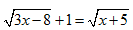3.5 In addition to Score 3.0 performance, the student demonstrates in-depth inferences and applications regarding the more complex content with partial success. 3.0 (proficient) The student can: solve a radical equation and identify an extraneous solution. provide examples of how extraneous solutions to a radical equation arise. The student exhibits no major errors or omissions. Solve the following equations: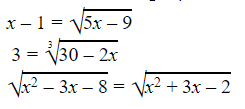2.5 The student demonstrates no major errors or omissions regarding the simpler details and processes (Score 2.0 content) and partial knowledge of the more complex ideas and processes (Score 3.0 content). 2.0 (progressing) There are no major errors or omissions regarding the simpler details and processes as the student can: recognize and recall specific terminology such as: radical radicand index inverse operations extraneous solution identify an extraneous solution to a radical equation when given possible solutions.      However, the student exhibits major errors or omissions regarding the more complex ideas and processes. Solve the following equations: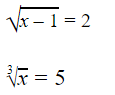1.5 The student demonstrates partial knowledge of the simpler details and processes (Score 2.0 content) but exhibits major errors or omissions regarding the more complex ideas and procedures (Score 3.0 content). 1.0 (beginning) With help, the student demonstrates a partial understanding of some of the simpler details and processes (Score 2.0 content) and some of the more complex ideas and processes (Score 3.0 content). 0.5 With help, the student demonstrates a partial understanding of some of the simpler details and processes (Score 2.0 content) but not the more complex ideas and processes (Score 3.0 content). 0.0 Even with help, the student demonstrates no understanding or skill.

## Alg II Solve Rational Equations Proficiency Scale

Score   Description Sample Activity

4.0

In addition to Score 3.0, the student demonstrates in-depth inferences and applications regarding more complex material that go beyond end of instruction expectations.

 Describe the error in the following work: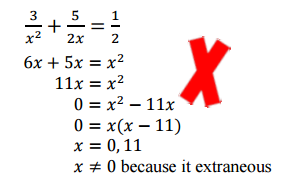3.5 In addition to Score 3.0 performance, the student demonstrates in-depth inferences and applications regarding the more complex content with partial success.

3.0

(proficient)

The student can:
• solve a rational equation and recognize an extraneous solution.
The student exhibits no major errors or omissions.

Solve the following rational equation for r  and check for extraneous solutions: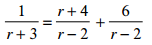2.5 The student demonstrates no major errors or omissions regarding the simpler details and processes (Score 2.0 content) and partial knowledge of the more complex ideas and processes (Score 3.0 content).

2.0

(progressing)

There are no major errors or omissions regarding the simpler details and processes as the student can:
• recognize and recall specific terminology such as:
• rational equation
• denominator
• extraneous solution
• solve simple rational equations.
However, the student exhibits major errors or omissions regarding the more complex ideas and processes.

Solve the following rational equation for n: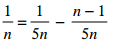1.5 The student demonstrates partial knowledge of the simpler details and processes (Score 2.0 content) but exhibits major errors or omissions regarding the more complex ideas and procedures (Score 3.0 content).

1.0

(beginning)

With help, the student demonstrates a partial understanding of some of the simpler details and processes (Score 2.0 content) and some of the more complex ideas and processes (Score 3.0 content).
0.5 With help, the student demonstrates a partial understanding of some of the simpler details and processes (Score 2.0 content) but not the more complex ideas and processes (Score 3.0 content).
0.0 Even with help, the student demonstrates no understanding or skill.

## Resources

 Web Vocab

#### MAT-HS.A-REI.03

 MAT-HS Targeted Standards(A) Concept: Algebra(REI) Domain: Reasoning with Equations and InequalitiesCluster: Understand solving equations as a process of reasoning and explain the reasoning MAT-HS.A-REI.03 Solve linear equations and inequalities in one variable, including equations with coefficients represented by letters. .

## Student Learning Targets:

• I can
• I can

• I can
• I can

### Skills (Performance) Targets

• I can solve for (isolate) a specific variable.
• I can perform transformations that produce equivalent equations (e.g., adding the same amount to both sides of the equation, etc.).
• I can check the solution.
• I can set a polynomial equal to zero and solve by factoring, quadratic formula, graphing, linear combinations, and substitution.
• I can solve for (isolate) a specific variable.
• I can write a linear system.
• I can perform transformations that produce equivalent equations (e.g., adding the same amount to both sides of the equation, etc.)
• I can check the solution.
• I can solve a quadratic.
• I can multiply and factor polynomials.
• I can solve a linear inequality.
• I can graph a linear inequality.
• I can solve a system of linear inequalities.
• I can graph a system of linear inequalities.
• I can check the solution of the system.
• I can solve a linear system (substitution, linear combination, graphically).
• I can write a linear system.
• I can identify the number of solutions of a linear system.
• I can identify the most efficient method to solve a system.
• I can check the solution of the system.
• I can solve linear equations in one variable, including equations with coefficients represented by letters**.
• I can solve linear inequalities in one variable, including inequalities with coefficients represented by letters**.

• I can
• I can

## Proficiency Scale

 Score Description Sample Activity 4.0 In addition to achieving level 3.0 content, the student makes in-depth inferences and applications that go beyond what was taught |3c+4|=6       |x+3|+2<5 3.5 In addition to Score 3.0 performance, the student demonstrates in-depth inferences and applications regarding the more complex content with partial success. 3.0 The student can: o solves linear equations with one variable:     -Additive Inverse     -Multiplicative Inverse     -Distributive Property     -Combine like terms     -Proportions **including special cases of no solution, infinitely many solutions o solve and graph the solution set of linear inequalities with one variable:     -Apply the properties of linear equation     -Multiplicative Inverse of negative coefficients The student exhibits no major errors or omissions. o  3(h-4)=-1224-6h+2h o  52c-3=37c+4 o  -67y-642 o  Solve for x: ax+bc=d o  -4 13 2.5 The student demonstrates no major errors or omissions regarding the simpler details and processes (Score 2.0 content) and partial knowledge of the more complex ideas and processes (Score 3.0 content). 2.0 There are no major errors or omissions regarding the simpler details and processes as the student: o  demonstrates basics processes (HS.A-REI.01)     -one-step equations/inequalities     -two-step equations/inequalities However, the student exhibits major errors or omissions regarding the more complex ideas and processes. o  3x=14 o  -3x+5-2x=10 o  x2=83 o  4x-17 o  Solve for x: x+y=c 1.5 The student demonstrates partial knowledge of the simpler details and processes (Score 2.0 content) but exhibits major errors or omissions regarding the more complex ideas and procedures (Score 3.0 content). 1.0 With help, a partial understanding of some of the simpler details and processes and some of the more complex ideas and processes. - 0.5 With help, the student demonstrates a partial understanding of some of the simpler details and processes (Score 2.0 content) but not the more complex ideas and processes (Score 3.0 content).

## Resources

 Web Vocab

#### MAT-HS.A-REI.03a

 MAT-HS Targeted Standards(A) Concept: Algebra(REI) Domain: Reasoning with Equations and InequalitiesCluster: Understand solving equations as a process of reasoning and explain the reasoning MAT-HS.A-REI.03a Solve linear equations in one variable, including equations with coefficients represented by letters. .

## Student Learning Targets:

• I can
• I can

• I can
• I can

### Skills (Performance) Targets

• I can solve for (isolate) a specific variable.
• I can perform transformations that produce equivalent equations (e.g., adding the same amount to both sides of the equation, etc.).
• I can check the solution.
• I can set a polynomial equal to zero and solve by factoring, quadratic formula, graphing, linear combinations, and substitution.
• I can solve for (isolate) a specific variable.
• I can write a linear system.
• I can perform transformations that produce equivalent equations (e.g., adding the same amount to both sides of the equation, etc.)
• I can check the solution.
• I can solve a quadratic.
• I can multiply and factor polynomials.
• I can solve a linear inequality.
• I can graph a linear inequality.
• I can solve a system of linear inequalities.
• I can graph a system of linear inequalities.
• I can check the solution of the system.
• I can solve a linear system (substitution, linear combination, graphically).
• I can write a linear system.
• I can identify the number of solutions of a linear system.
• I can identify the most efficient method to solve a system.
• I can check the solution of the system.
• I can solve linear equations in one variable, including equations with coefficients represented by letters**.
• I can solve linear inequalities in one variable, including inequalities with coefficients represented by letters**.

• I can
• I can

## Proficiency Scale

 Score Description Sample Activity 4.0 In addition to achieving level 3.0 content, the student makes in-depth inferences and applications that go beyond what was taught |3c+4|=6       |x+3|+2<5 3.5 In addition to Score 3.0 performance, the student demonstrates in-depth inferences and applications regarding the more complex content with partial success. 3.0 The student can: o solves linear equations with one variable:     -Additive Inverse     -Multiplicative Inverse     -Distributive Property     -Combine like terms     -Proportions **including special cases of no solution, infinitely many solutions o solve and graph the solution set of linear inequalities with one variable:     -Apply the properties of linear equation     -Multiplicative Inverse of negative coefficients The student exhibits no major errors or omissions. o  3(h-4)=-1224-6h+2h o  52c-3=37c+4 o  -67y-642 o  Solve for x: ax+bc=d o  -4 13 2.5 The student demonstrates no major errors or omissions regarding the simpler details and processes (Score 2.0 content) and partial knowledge of the more complex ideas and processes (Score 3.0 content). 2.0 There are no major errors or omissions regarding the simpler details and processes as the student: o  demonstrates basics processes (HS.A-REI.01)     -one-step equations/inequalities     -two-step equations/inequalities However, the student exhibits major errors or omissions regarding the more complex ideas and processes. o  3x=14 o  -3x+5-2x=10 o  x2=83 o  4x-17 o  Solve for x: x+y=c 1.5 The student demonstrates partial knowledge of the simpler details and processes (Score 2.0 content) but exhibits major errors or omissions regarding the more complex ideas and procedures (Score 3.0 content). 1.0 With help, a partial understanding of some of the simpler details and processes and some of the more complex ideas and processes. - 0.5 With help, the student demonstrates a partial understanding of some of the simpler details and processes (Score 2.0 content) but not the more complex ideas and processes (Score 3.0 content).

## Resources

 Web Vocab

#### MAT-HS.A-REI.03b

 MAT-HS Targeted Standards(A) Concept: Algebra(REI) Domain: Reasoning with Equations and InequalitiesCluster: Understand solving equations as a process of reasoning and explain the reasoning MAT-HS.A-REI.03b Solve linear inequalities in one variable, including equations with coefficients represented by letters. .

## Student Learning Targets:

• I can
• I can

• I can
• I can

### Skills (Performance) Targets

• I can solve for (isolate) a specific variable.
• I can perform transformations that produce equivalent equations (e.g., adding the same amount to both sides of the equation, etc.).
• I can check the solution.
• I can set a polynomial equal to zero and solve by factoring, quadratic formula, graphing, linear combinations, and substitution.
• I can solve for (isolate) a specific variable.
• I can write a linear system.
• I can perform transformations that produce equivalent equations (e.g., adding the same amount to both sides of the equation, etc.)
• I can check the solution.
• I can solve a quadratic.
• I can multiply and factor polynomials.
• I can solve a linear inequality.
• I can graph a linear inequality.
• I can solve a system of linear inequalities.
• I can graph a system of linear inequalities.
• I can check the solution of the system.
• I can solve a linear system (substitution, linear combination, graphically).
• I can write a linear system.
• I can identify the number of solutions of a linear system.
• I can identify the most efficient method to solve a system.
• I can check the solution of the system.
• I can solve linear equations in one variable, including equations with coefficients represented by letters**.
• I can solve linear inequalities in one variable, including inequalities with coefficients represented by letters**.

• I can
• I can

## Proficiency Scale

 Score Description Sample Activity 4.0 In addition to achieving level 3.0 content, the student makes in-depth inferences and applications that go beyond what was taught |3c+4|=6       |x+3|+2<5 3.5 In addition to Score 3.0 performance, the student demonstrates in-depth inferences and applications regarding the more complex content with partial success. 3.0 The student can: o solves linear equations with one variable:     -Additive Inverse     -Multiplicative Inverse     -Distributive Property     -Combine like terms     -Proportions **including special cases of no solution, infinitely many solutions o solve and graph the solution set of linear inequalities with one variable:     -Apply the properties of linear equation     -Multiplicative Inverse of negative coefficients The student exhibits no major errors or omissions. o  3(h-4)=-1224-6h+2h o  52c-3=37c+4 o  -67y-642 o  Solve for x: ax+bc=d o  -4 13 2.5 The student demonstrates no major errors or omissions regarding the simpler details and processes (Score 2.0 content) and partial knowledge of the more complex ideas and processes (Score 3.0 content). 2.0 There are no major errors or omissions regarding the simpler details and processes as the student: o  demonstrates basics processes (HS.A-REI.01)     -one-step equations/inequalities     -two-step equations/inequalities However, the student exhibits major errors or omissions regarding the more complex ideas and processes. o  3x=14 o  -3x+5-2x=10 o  x2=83 o  4x-17 o  Solve for x: x+y=c 1.5 The student demonstrates partial knowledge of the simpler details and processes (Score 2.0 content) but exhibits major errors or omissions regarding the more complex ideas and procedures (Score 3.0 content). 1.0 With help, a partial understanding of some of the simpler details and processes and some of the more complex ideas and processes. - 0.5 With help, the student demonstrates a partial understanding of some of the simpler details and processes (Score 2.0 content) but not the more complex ideas and processes (Score 3.0 content).

## Resources

 Web Vocab

#### MAT-HS.A-REI.04

 MAT-HS Targeted Standards(A) Concept: Algebra(REI) Domain: Reasoning with Equations and InequalitiesCluster: Understand solving equations as a process of reasoning and explain the reasoning MAT-HS.A-REI.04  Solve quadratic equations in one variable.   a.  Use the method of completing the square to transform any quadratic equation in x into an equation of the form (x – p)² = q that has the same solutions.   (+) Derive the quadratic formula from this form.   b.  Solve quadratic equations by inspection (e.g., for x² = 49), taking square roots, completing the square, the quadratic formula and factoring, as appropriate to the initial form of the equation. Recognize when the quadratic formula gives complex solutions and write them as a ± bi for real numbers a and b.

## Student Learning Targets:

• I can
• I can

### Reasoning Targets

• I can explain why taking the square root of both sides of an equation can yield two solutions.

### Skills (Performance) Targets

• I can solve for (isolate) a specific variable.
• I can perform transformations that produce equivalent equations (e.g., adding the same amount to both sides of the equation, etc.).
• I can check the solution.
• I can solve for (isolate) a specific variable.
• I can solve a quadratic.
• I can multiply and factor polynomials.
• I can solve quadratic equations in one variable.
• I can transform a quadratic equation to an equation in the form (x-p)2=q by completing the square.
• I can derive the quadratic formula by completing the square on a quadratic equation.
• I can solve quadratic equations in one variable by simple inspection, taking the square root, factoring, and completing the square.
• I can use the quadratic formula to solve any quadratic equation, recognizing the formula produces all complex solutions and write the solutions in the form a ± bi , where a and b are real numbers.

• I can
• I can

## Algebra II Solving Quadratic Equations Proficiency Scale

 Score Description Sample Activity 4.0 In addition to Score 3.0, the student demonstrates in-depth inferences and applications regarding more complex material that go beyond end of instruction expectations. Suppose the quadratic functions f(x)=ax2+bx+c with f(-2)=0 and -b/(2a) =1. Solve f(x)=0. 3.5 In addition to Score 3.0 performance, the student demonstrates in-depth inferences and applications regarding the more complex content with partial success. 3.0 The student can: solve a quadratic equation using any method. Solve the following quadratic equation using any method: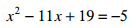x2+4x+15=0 2.5 The student demonstrates no major errors or omissions regarding the simpler details and processes (Score 2.0 content) and partial knowledge of the more complex ideas and processes (Score 3.0 content). 2.0 There are no major errors or omissions regarding the simpler details and processes as the student can: solve simple quadratic equations. recognize and recalls specific terminology, such as: quadratic equation factor complete the square quadratic formula square root imaginary/complex numbers Solve the following quadratic equations: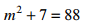x2+100=0 Perform the given operation: (3+i)(-6-i) 1.5 The student demonstrates partial knowledge of the simpler details and processes (Score 2.0 content) but exhibits major errors or omissions regarding the more complex ideas and procedures (Score 3.0 content). 1.0 With help, the student demonstrates a partial understanding of some of the simpler details and processes (Score 2.0 content) and some of the more complex ideas and processes (Score 3.0 content). - 0.5 With help, the student demonstrates a partial understanding of some of the simpler details and processes (Score 2.0 content) but not the more complex ideas and processes (Score 3.0 content). 0.0 Even with help, the student demonstrates no understanding or skill. -

## Resources

 Web Vocab completing the square quadratic formula

#### MAT-HS.A-REI.04.b

 MAT-HS Targeted Standards(A) Concept: Algebra(REI) Domain: Reasoning with Equations and InequalitiesCluster: Understand solving equations as a process of reasoning and explain the reasoning MAT-HS.A-REI.04.b Solve quadratic equations in one variable. b. Solve quadratic equations by inspection (e.g., for x²= 49), taking square roots, completing the square, the quadratic formula and factoring, as appropriate to the initial form of the equation. Recognize when the quadratic formula gives complex solutions and write them as a ± bi for real numbers a and b.

## Student Learning Targets:

• I can
• I can

### Reasoning Targets

• I can explain why taking the square root of both sides of an equation can yield two solutions.

### Skills (Performance) Targets

• I can solve quadratic equations by using square roots.
• I can solve quadratic equations by factoring.
• I can solve quadratic equations by completing the square.
• I can find the number of solutions of a quadratic equation.

• I can
• I can

## Proficiency Scale

 Score Description Sample Activity 4.0 In addition to achieving level 3.0 content, the student makes in-depth inferences and applications that go beyond what was taught Use the method of completing the square to derive the quadratic formula. Solve a quadratic equation that has a complex solution and write in the form a + bi 3.5 In addition to Score 3.0 performance, the student demonstrates in-depth inferences and applications regarding the more complex content with partial success. 3.0 The student can: Solve quadratic equations by: using square roots factoring using the quadratic formula (include equations with zero, one, or two real-solutions) The student exhibits no major errors or omissions. Solve the quadratic equation by finding square roots.            3x2 - 12 = 0 Solve the quadratic equation by factoring.         x2 - 14x + 45 = 0 Use the Quadratic formula to solve the equation.           5x2 - 13x  =  -9 2.5 The student demonstrates no major errors or omissions regarding the simpler details and processes (Score 2.0 content) and partial knowledge of the more complex ideas and processes (Score 3.0 content). 2.0 There are no major errors or omissions regarding the simpler details and processes as the student: However, the student exhibits major errors or omissions regarding the more complex ideas and processes. x2 = 49 (4t + 1)(t - 2) = 0 Identify a, b, and c as it’s used in the quadratic formula x2 + 6x = - 5 1.5 The student demonstrates partial knowledge of the simpler details and processes (Score 2.0 content) but exhibits major errors or omissions regarding the more complex ideas and procedures (Score 3.0 content). 1.0 With help, a partial understanding of some of the simpler details and processes and some of the more complex ideas and processes. - 0.5 With help, the student demonstrates a partial understanding of some of the simpler details and processes (Score 2.0 content) but not the more complex ideas and processes (Score 3.0 content).

## Resources

 Web Vocab completing the square quadratic formula

#### MAT-HS.A-REI.05

 MAT-HS Targeted Standards(A) Concept: Algebra(REI) Domain: Reasoning with Equations and InequalitiesCluster: Solve systems of equations MAT-HS.A-REI.05 Prove that, given a system of two equations in two variables, replacing one equation by the sum of that equation and a multiple of the other produces a system with the same solutions.

## Student Learning Targets:

• I can
• I can

• I can
• I can

### Skills (Performance) Targets

• I can solve for (isolate) a specific variable.
• I can perform transformations that produce equivalent equations (e.g., adding the same amount to both sides of the equation, etc.).
• I can check the solution.
• I can set a polynomial equal to zero and solve by factoring, quadratic formula, graphing, linear combinations, and substitution.
• I can solve for (isolate) a specific variable.
• I can write a linear system.
• I can perform transformations that produce equivalent equations (e.g., adding the same amount to both sides of the equation, etc.)
• I can check the solution.
• I can solve a quadratic.
• I can multiply and factor polynomials.
• I can solve a linear inequality.
• I can graph a linear inequality.
• I can solve a system of linear inequalities.
• I can graph a system of linear inequalities.
• I can check the solution of the system.
• I can solve a linear system (substitution, linear combination, graphically).
• I can write a linear system.
• I can identify the number of solutions of a linear system.
• I can identify the most efficient method to solve a system.
• I can check the solution of the system.
• I can produce, with justification, from a system of two equations an equivalent simpler system.

• I can
• I can

## Proficiency Scale

 Score Description Sample Activity 4.0 In addition to Score 3.0, the student demonstrates in-depth inferences and applications regarding more complex material that go beyond end of instruction expectations. - 3.5 In addition to Score 3.0 performance, the student demonstrates in-depth inferences and applications regarding the more complex content with partial success. 3.0 “The Standard.” The student demonstrates no major errors or omissions regarding any of the information and processes that were end of instruction expectations. - 2.5 The student demonstrates no major errors or omissions regarding the simpler details and processes (Score 2.0 content) and partial knowledge of the more complex ideas and processes (Score 3.0 content). 2.0 The student demonstrates no major errors or omissions regarding the simpler details and processes but exhibits major errors or omissions regarding the more complex ideas and processes (Score 3.0 content). - 1.5 The student demonstrates partial knowledge of the simpler details and processes (Score 2.0 content) but exhibits major errors or omissions regarding the more complex ideas and procedures (Score 3.0 content). 1.0 With help, the student demonstrates a partial understanding of some of the simpler details and processes (Score 2.0 content) and some of the more complex ideas and processes (Score 3.0 content). - 0.5 With help, the student demonstrates a partial understanding of some of the simpler details and processes (Score 2.0 content) but not the more complex ideas and processes (Score 3.0 content). 0.0 Even with help, the student demonstrates no understanding or skill. -

## Resources

 Web Vocab

Page: (Previous)   1  2  3  4  5  (Next)
ALL